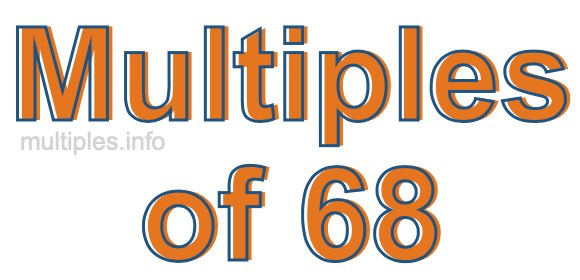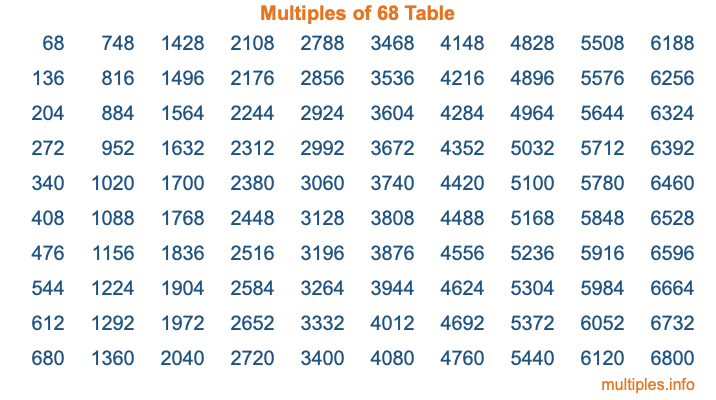Multiples of 68Welcome to the Multiples of 68 page. Here we will first teach you everything you will ever need to know about the multiples of 68, and then give you a study guide summary of everything we taught you to make sure you remember it all. Use this page to look up facts and learn information about the multiples of 68. This page will make you a multiples of sixty-eight expert!

Definition of Multiples of 68
Multiples of 68 are all the numbers that when divided by 68 equal an integer. Each of the multiples of 68 are called a multiple. A multiple of 68 is created by multiplying 68 by an integer.

Therefore, to create a list of multiples of 68, you start with 1 multiplied by 68, then 2 multiplied by 68, then 3 multiplied by 68, and so on for as long as you want. Thus, the list of the first five multiples of 68 is 68, 136, 204, 272, and 340. To see a larger list of multiples of 68, see the printable image of Multiples of 68 further down on this page. We also have a category where you can choose any nth multiple of 68.

Multiples of 68 Checker
The Multiples of 68 Checker below checks to see if any number of your choice is a multiple of 68. In other words, it checks to see if there is any number (integer) that when multiplied by 68 will equal your number. To do that, we divide your number by 68. If the the quotient is an integer, then your number is a multiple of 68.

Is  a multiple of 68?

Least Common Multiple of 68 and ...
A Least Common Multiple (LCM) is the lowest multiple that two or more numbers have in common. This is also called the smallest common multiple or lowest common multiple and is useful to know when you are adding our subtracting fractions. Enter one or more numbers below (68 is already entered) to find the LCM.

Check out our LCM Calculator if you need more details about the Least Common Multiple or if you need the LCM for different numbers for adding and subtraction fractions.

nth Multiple of 68
As we stated above, 68 is the first multiple of 68, 136 is the second multiple of 68, 204 is the third multiple of 68, and so on. Enter a number below to find the nth multiple of 68.

th multiple of 68

Multiples of 68 vs Factors of 68
68 is a multiple of 68 and a factor of 68, but that is where the similarities end. All postive multiples of 68 are 68 or greater than 68. All positive factors of 68 are 68 or less than 68.

Below is the beginning list of multiples of 68 and the factors of 68 so you can compare:

Multiples of 68: 68, 136, 204, 272, 340, etc.

Factors of 68: 1, 2, 4, 17, 34, 68

As you can see, the multiples of 68 are all the numbers that you can divide by 68 to get a whole number. The factors of 68, on the other hand, are all the whole numbers that you can multiply by another whole number to get 68.

It's also interesting to note that if a number (x) is a factor of 68, then 68 will also be a multiple of that number (x).

Multiples of 68 vs Divisors of 68
The divisors of 68 are all the integers that 68 can be divided by evenly. Below is a list of the divisors of 68.

Divisors of 68: 1, 2, 4, 17, 34, 68

The interesting thing to note here is that if you take any multiple of 68 and divide it by a divisor of 68, you will see that the quotient is an integer.

Multiples of 68 Table
Below is an image of the first 100 multiples of 68 in a table. The table is in chronological order, column by column. The first column has the first ten multiples of 68, the second column has the next ten multiples of 68, and so on.The Multiples of 68 Table is also referred to as the 68 Times Table or Times Table of 68. You are welcome to print out our table for your studies.

Negative Multiples of 68
Although not often discussed or needed in math, it is worth mentioning that you can make a list of negative multiples of 68 by multiplying 68 by -1, then by -2, then by -3, and so on, to get the following list of negative multiples of 68:

-68, -136, -204, -272, -340, etc.

Multiples of 68 Summary
Below is a summary of important Multiples of 68 facts that we have discussed on this page. To retain the knowledge on this page, we recommend that you read through the summary and explain to yourself or a study partner why they hold true.

There are an infinite number of multiples of 68.

A multiple of 68 divided by 68 will equal a whole number.

68 divided by a factor of 68 equals a divisor of 68.

The nth multiple of 68 is n times 68.

The largest factor of 68 is equal to the first positive multiple of 68.

68 is a multiple of every factor of 68.

68 is a multiple of 68.

A multiple of 68 divided by a divisor of 68 equals an integer.

68 divided by a divisor of 68 equals a factor of 68.

Any integer times 68 will equal a multiple of 68.

Multiples of a Number
Here you can get the multiples of another number, all with the same attention to detail as we did for multiples of 68 on this page.

Multiples of
Multiples of 69
Did you find our page about multiples of sixty-eight educational? Do you want more knowledge? Check out the multiples of the next number on our list!